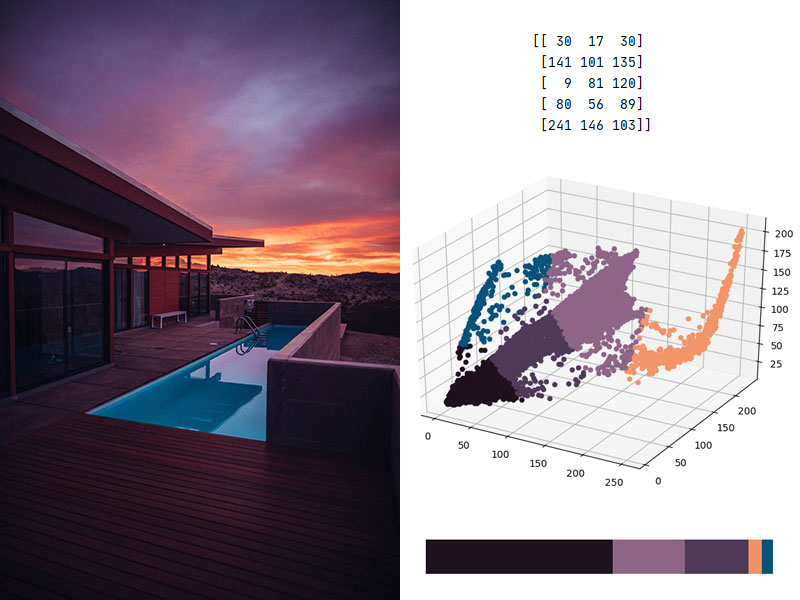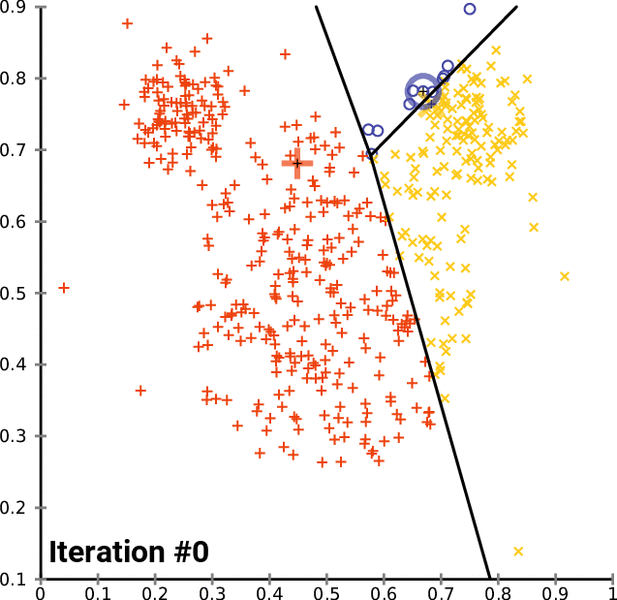## Finding the dominant colors in an image with k-means

Working with images can be a very time-consuming task, especially if you have many images to work on. Machine learning can thus be a great time-saver for various image analysis and editing tasks, such as finding the dominant colors of an image thanks to the K-means clustering algorithm.## How to program the K-Means clustering algorithm

K-Means is a popular unsupervised machine learning algorithm for data clustering. A typical start for flat clustering, the K-Means algorithm works by defining a number K of clusters to be extracted by the algorithm. With this K number given, the algorithm will then find the best “centroids” to cluster the data around.Continue reading “How to program the K-Means clustering algorithm”